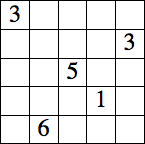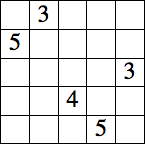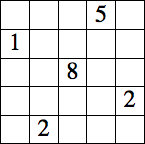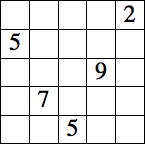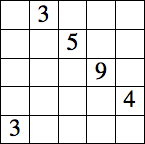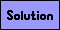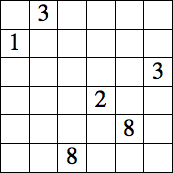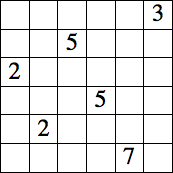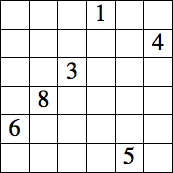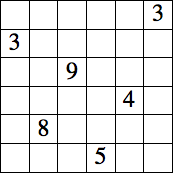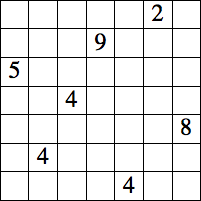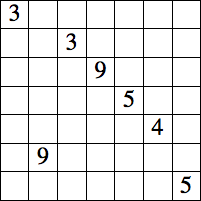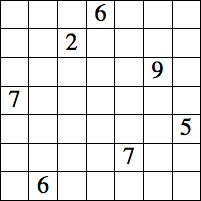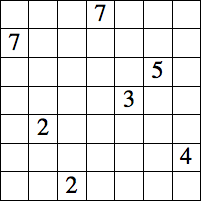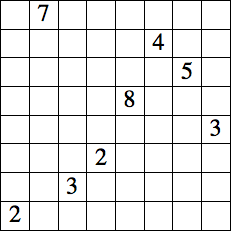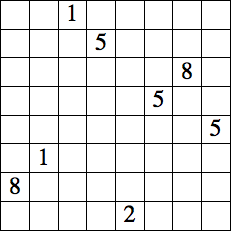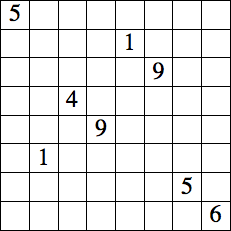Middle Sum Puzzles

Fill in some of the blank squares with digits 1-9 so that each row and column contains exactly 3 digits, with the middle number in each row and column being the sum of the other two. A sample puzzle is shown below.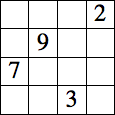→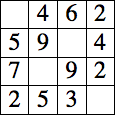Each puzzle below has a unique solution.